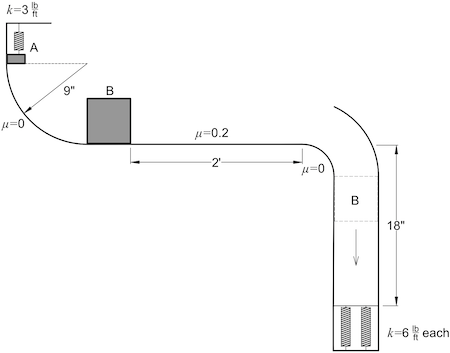# Block A, weight 2 lbs, is pushed up against a spring (k=3lb/ft) compressing it 6 inches in the...

## Question:

Block A, weight 2 lbs, is pushed up against a spring ({eq}k=3\frac{\text{lb}}{\text{ft}} {/eq}) compressing it 6 inches in the position shown. Block A slides on a smooth curve of 9 inch radius and collides with block B, weight 3 lbs, and initially at rest. After the impact, block B is observed to slide to the right on a horizontal surface with a coefficient of friction of 0.2. At the end of the horizontal surface, block B drops 18 inches and compresses two springs, each with a stiffness of {eq}k=6\frac{\text{lb}}{\text{ft}} {/eq}. The coefficient of restitution between A and B is 0.6. Neglect all impacts after A and B.

Find:

a.) velocity of A before it strikes B

b.) velocity of A and B after the impact

c.) speed of b and the end of the horizontal surface

d.) amount the equivalent spring is compressed by the fall of block B

e.) how high block B rebounds## Determining Height For Bouncing Object:

For a scenario where an object is in motion and collides with a plane in such a way that the object bounces back the height of the object is calculated using the energy conservation and the final velocity is calculated by means of the work-energy theorem.

y= 6 in = 0.5 ft

h = 9 in=0.75 ft

(a) Using work energy thorem

{eq}\displaystyle W=\frac{ky^2}{2}\\ W=3 lb/ft(\frac{0.5 \ ft}{2})^2=0.375 \ lb.ft {/eq}

Using energy conservation

{eq}\displaystyle 0.375+2(0.75)=\frac{m_AV_A^2}{2}\\ m_A=\frac{W_A}{g}=\frac{2}{32.18}=0.062 \ slug\\ m_B=\frac{W_B}{g}=\frac{3}{32.18}=0.093 \ slug\\ 0.375+2(0.75)=\frac{0.0625 V_A^2}{2}\\ V_A=7.76 ft/s {/eq}

(b) {eq}\displaystyle m_AV_A+m_BV_B=m_AV_A(f)+m_BV_B(f)\\ 0.062\times 7.76=0.062 V_A(f)+0.093 V_B(f)\\ 0.4811=0.062V_A(f)+0.093V_B(f)\\ \frac{-(V_A(f)-V_B(f))}{V_A-V_B}=0.6\\ \frac{-(V_A(f)-V_B(f))}{7.76}=0.6\\ -V_A(f)+V_B(f)=4.656 {/eq}

Solving the equations we get final velocities as

{eq}\displaystyle V_A(f)=0.31 \ ft/s\\ V_B(f)=4.97 \ ft/s {/eq}

(c) {eq}\displaystyle V_B^2(f)=V_B^2+2ax\\ a<0\\ m_Ba=-F_R=-\mu N=-\mu W_B=-0.2\times 3=-0.6 \ lb\\ a=\frac{-0.6}{0.0935}=-64.5 ft/s^2\\ x=2'=0.16 \ ft\\ V_B(f)=\sqrt V_B^2+2ax\\ =\sqrt (4.97^2+2(-64.5)(0.16))\\ V_B(f)=4.75 \ ft/s {/eq}

(d) h=1.5 ft

Using energy conservation

{eq}\displaystyle E_i=W_B\times h=3\times 1.5 = 4.5 lb.ft\\ V_B^2(f)=V_B(f)+2gh\\ V_B(f)=\sqrt{2gh}=\sqrt (2\times 32.18\times 1.5)=9.82 \ ft/s {/eq}

Final energy is {eq}\displaystyle E_f=\frac{m_BV_B^2(f)}{2}+\frac{k_1y^2}{2}+\frac{k_2y^2}{2}\\ k_1=k_2=6 lb/ft\\ E_f=\frac{0.093\text{slug}\times 9.8}{2}+6y^2\\ E_f=4.48+6y^2 {/eq}

So,

{eq}\displaystyle E_i=E_f\\ 4.5=4.48+6y^2\\ y=0.051 \ ft {/eq}